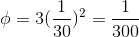# Calculus 1 : How to find approximation of rate

## Example Questions

### Example Question #1 : Approximation Of Rate

Find the average rate of change for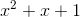over the interval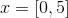.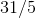Explanation:

The rate of change of a function is the amount it changes over a given amount of time.

In mathematical terms, this can be written as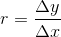we plug in our values: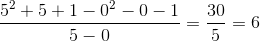Note that this is only an average because quadratic functions change at different rates depending on where you are in the function's domain.

### Example Question #2 : Approximation Of Rate

Let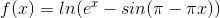. Use linear approximation to estimate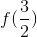.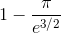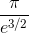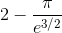Explanation:

Note that: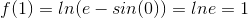Therefore, for values relatively close  to 1, we can use the formula for dy (the differential form of the derivative) to estimate f at close values.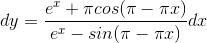From log derivative with chain rule.

Sinceliesto the right of,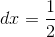andfor the estimation, so: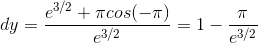So then: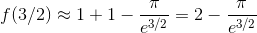### Example Question #3 : Approximation Of Rate

Using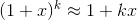, approximate the value of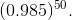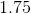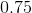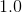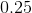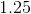Explanation:

First, we need to rearrange the given to match the approximation formula.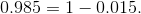Therefore,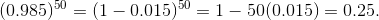### Example Question #4 : Approximation Of Rate

A cube is diminishing in size. What is the ratio of the rate of loss of the cube's volume to the rate of loss of its sides when its sides have length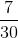?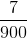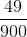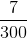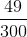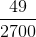Explanation:

Begin by writing the equations for a cube's dimensions. Namely its volume in terms of the length of its sides: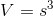The rates of change of the volume can be found by taking the derivative of each side of the equation with respect to time: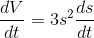Now, knowing the length of the sides, simply divide to find the ratio between the rate of change of the volume and sides: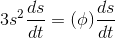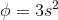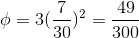### Example Question #5 : Approximation Of Rate

A cube is diminishing in size. What is the ratio of the rate of loss of the cube's volume to the rate of loss of its sides when its sides have length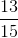?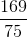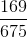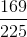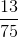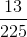Explanation:

Begin by writing the equations for a cube's dimensions. Namely its volume in terms of the length of its sides:The rates of change of the volume can be found by taking the derivative of each side of the equation with respect to time:Now, knowing the length of the sides, simply divide to find the ratio between the rate of change of the volume and sides: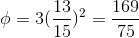### Example Question #6 : Approximation Of Rate

A cube is diminishing in size. What is the ratio of the rate of loss of the cube's volume to the rate of loss of its sides when its sides have length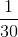?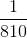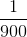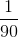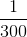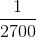Explanation:

Begin by writing the equations for a cube's dimensions. Namely its volume in terms of the length of its sides:The rates of change of the volume can be found by taking the derivative of each side of the equation with respect to time:Now, knowing the length of the sides, simply divide to find the ratio between the rate of change of the volume and sides: